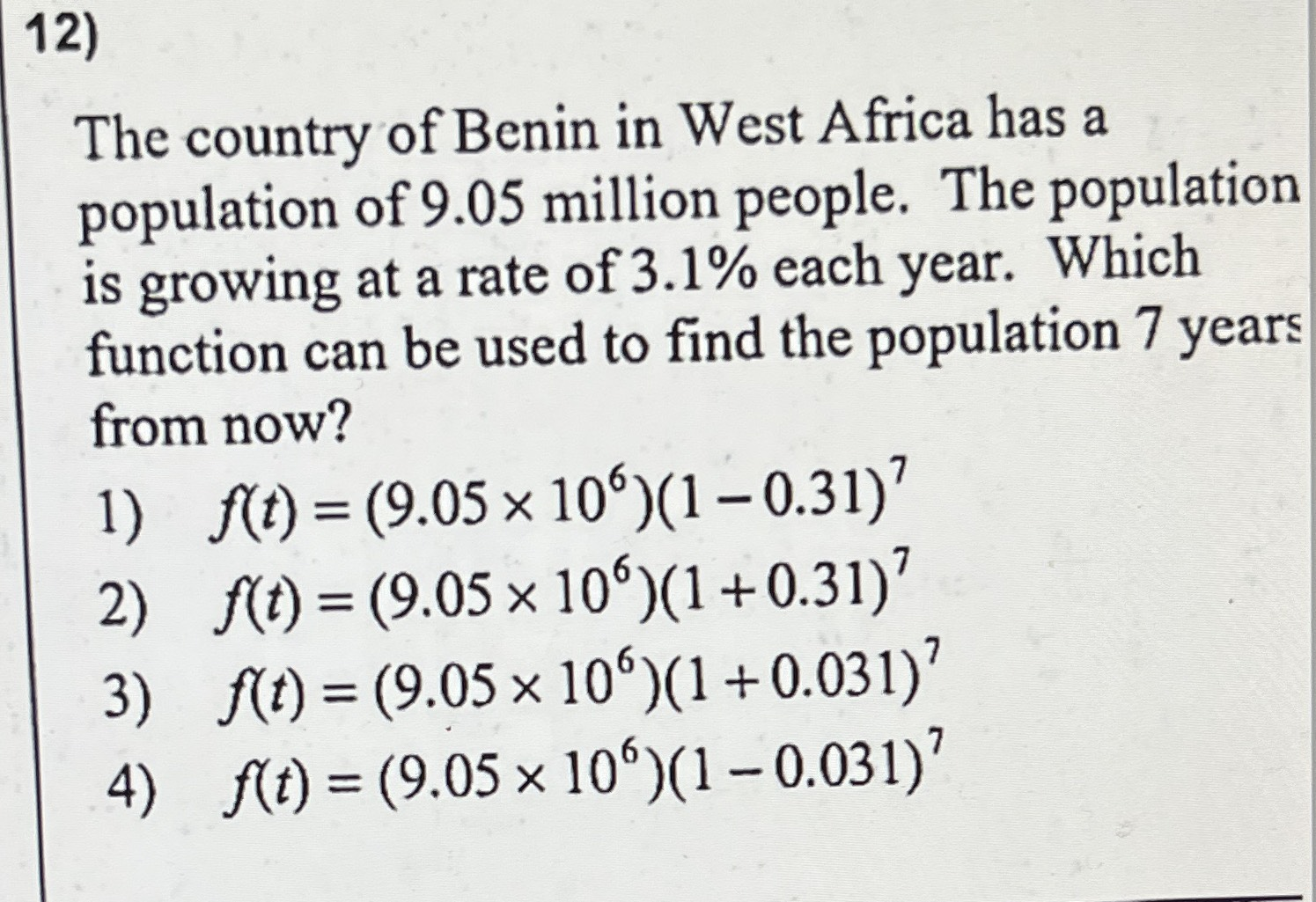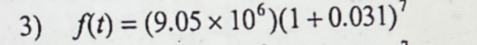### ¿Todavía tienes preguntas de matemáticas?

Pregunte a nuestros tutores expertos
Algebra
Pregunta12) The country of Benin in West Africa has a population of $$9.05$$ million people. The population is growing at a rate of $$3.1 \%$$ each year. Which function can be used to find the population $$7$$ years from now?

1) $$f ( t ) = ( 9.05 \times 10 ^ { 6 } ) ( 1 - 0.31 ) ^ { 7 }$$

2) $$f ( t ) = ( 9.05 \times 10 ^ { 6 } ) ( 1 + 0.31 ) ^ { 7 }$$

3) $$f ( t ) = ( 9.05 \times 10 ^ { 6 } ) ( 1 + 0.031 ) ^ { 7 }$$

4) $$f ( t ) = ( 9.05 \times 10 ^ { 6 } ) ( 1 - 0.031 ) ^ { 7 }$$